# -2- Compute Drift given Power and Bounds

If you haven't seen the vignette -1- Compute Bounds, it is recommended to visit this one first.

The option -2- menu initially looks as follows.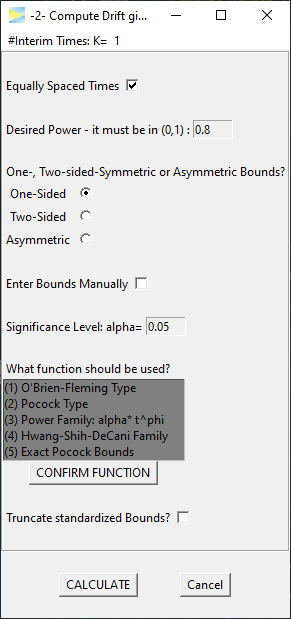### O'Brien-Fleming 3-stage design

For a start lets set K=3 stages and CALCULATE.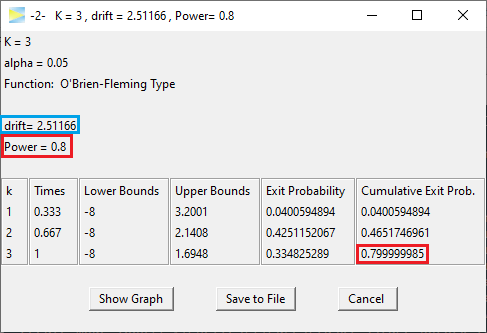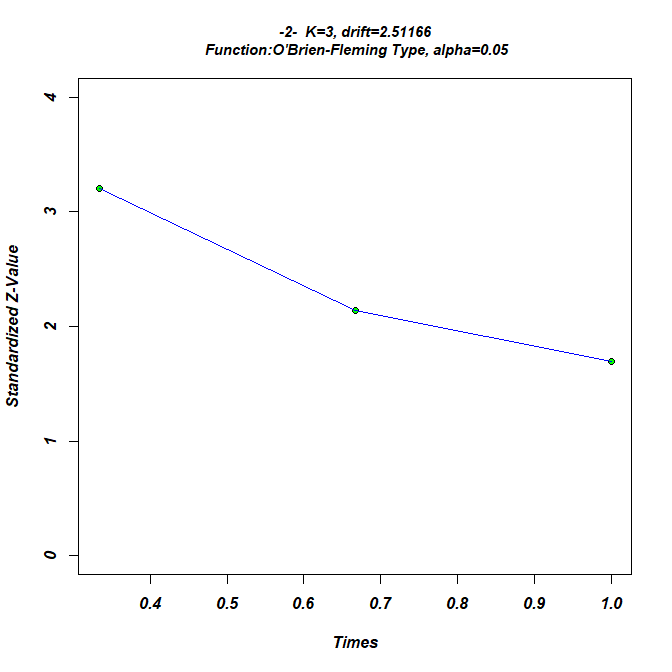The design maintains the power of 80%. The second to last column provides the Exit Probability for each stage. As is typical for O'Brien-Fleming designs, the probability to abort the study at the first interim look is very low (here 4%) even under H1. Then on the second stage, it's 42% and cumulative almost a 50% chance of having the study aborted by then and finally in one third of the cases the effect is detected at the last stage while in the remaining 20% of cases H0 will be accepted and the effect stays undetected. These probabilities are all valid if the true drift (i.e. true standardized effect size) is 2.51.

### Pocock 3-stage design

Next lets see the required drift if we use a Pocock design.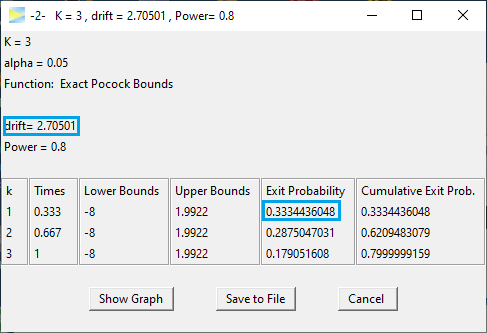Apparently, to achieve a power of 80%, this design requires an effect of 2.71, which is 8% higher than that of the O'Brien-Fleming designs. Comparing the power of both designs for a specific drift, is discussed in -3- Compute Probabilities given Bounds and Drift.

With the Pocock design on the other hand you will be able to abort the study at the first interim look in about 33% of the cases, so if you are optimistic about your expected effect, the Pocock design might be the better choice as it will result in lower required samples on average and thereby a faster study conclusion.

To change the desired power, just edit the value in the input window.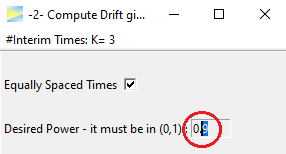If we re-CALCULATE, we get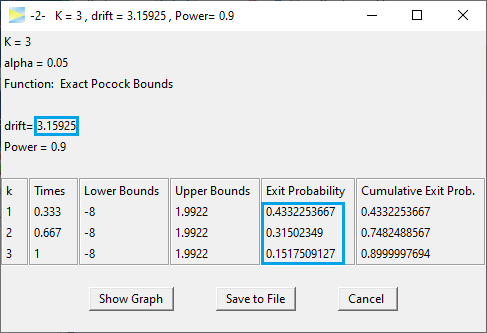As expected the required drift has increased to now 3.16, but there is also something interesting to the resulting exit probabilities as basically the 10% increase in power was "added" to the first interim look, which now has a probability of 43% to succeed. Also the exit probability at the final stage even has been decreased slightly.

First of all, this is good news, of course, because it means that it has become less likely that the full sample is required to come to a conclusion. Intuitively, this also makes sense, because a stronger effect indicated by the higher drift (on average) will be detected earlier than a weaker effect.

### Manual bounds

In order to enable the computation of drift for arbitrary designs, you can enter bounds manually.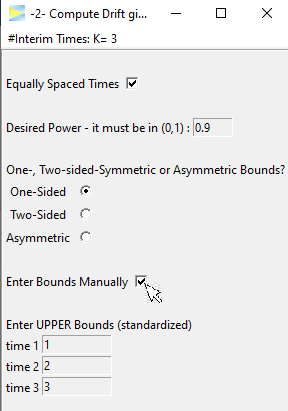Hitting CALCULATE for the default bounds 1, 2, 3, we get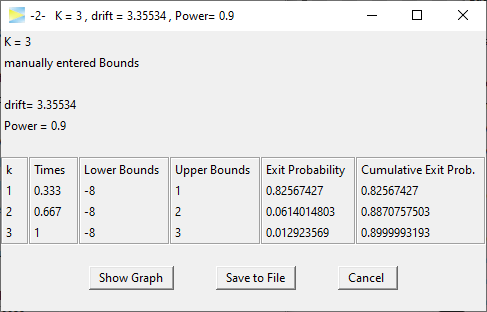This design maintains the specified power, if the drift was 3.355, but it does not maintain the 5% alpha level under H0 (drift = 0).

To see this, check out the next vignette -3- Compute Probabilities given Bounds and Drift